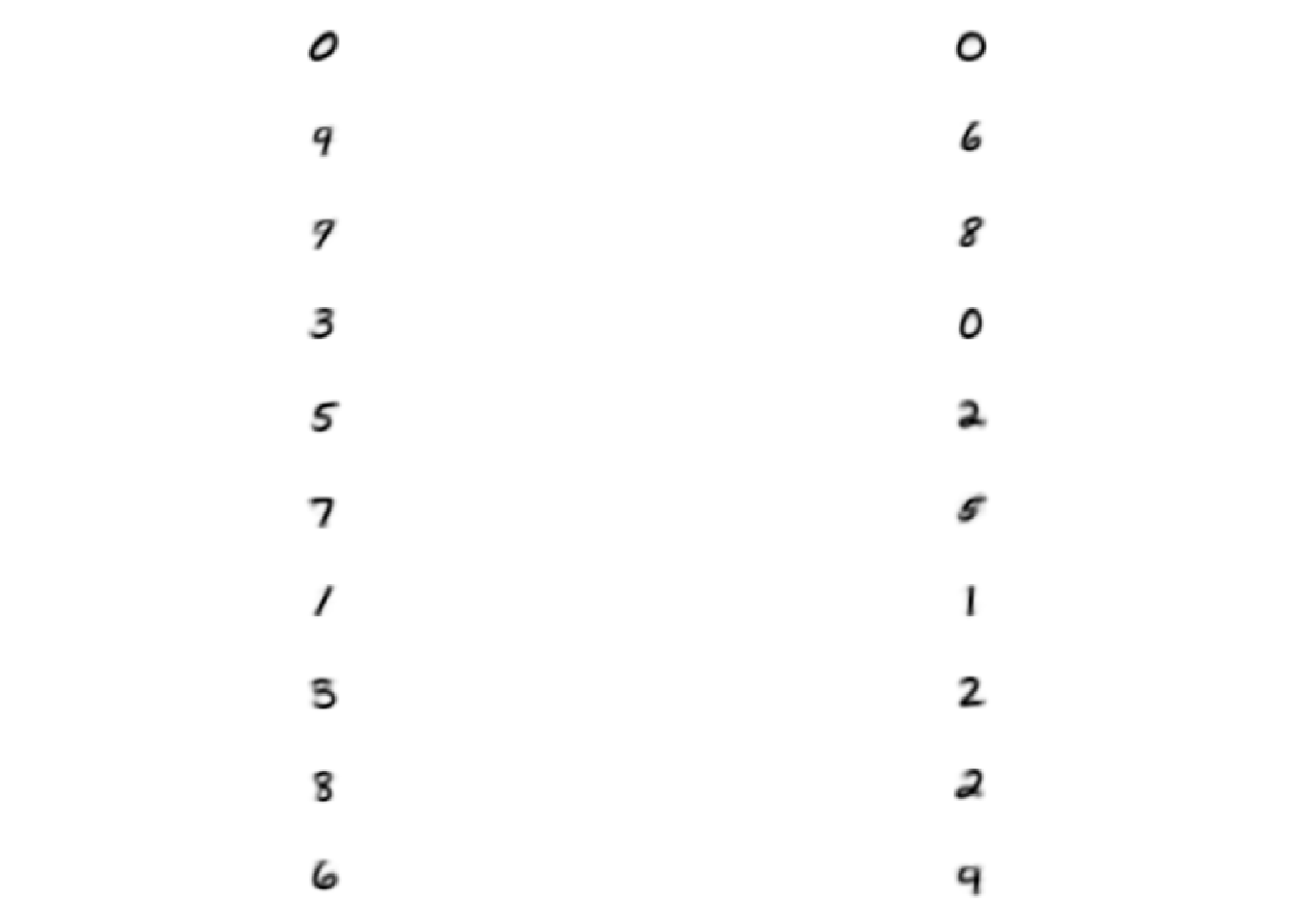## Dataset

I’m using the Digit Recognizer (MNIST) dataset

## Preparing the data

First we load the data from CSV files. I wrote a function to read and transform the data given a file name. Notice the cache=TRUE flag in the report. This means that as the report is changed, I don’t have to wait for the data to get reloaded.

trainFile <- "D:/Mnist/train.csv"
testFile <- ""

loadData <- function(fileName) {
data <- read.csv(fileName, header = TRUE, sep = ",")
data$label <- factor(data$label) #store lables as factor
data
}

trainData <- loadData(trainFile)

Next, I look at the names of the variables (the column names of the dataframe):

names(trainData)[1:10]
##   "label"  "pixel0" "pixel1" "pixel2" "pixel3" "pixel4" "pixel5"
##   "pixel6" "pixel7" "pixel8"
n <- length(colnames(trainData)) #length of column names list
imageSize <- sqrt(n - 1) #remove 1 because of the labels
imageSize
##  28
numCols <- dim(trainData) #number of columns
numCols
##  42000
#indexes of features excluding label column
trainFeatureIndexes <- which(!(names(trainData) %in% c("label")))

Looks good! We have 42000 images of 28 times 28 pixels. The label is an extra column which I transformed to factor.

Here is a histogram of the labels in the training data.

barplot(table(trainData$label), main="Labels in training data",xlab="label",ylab="frequency")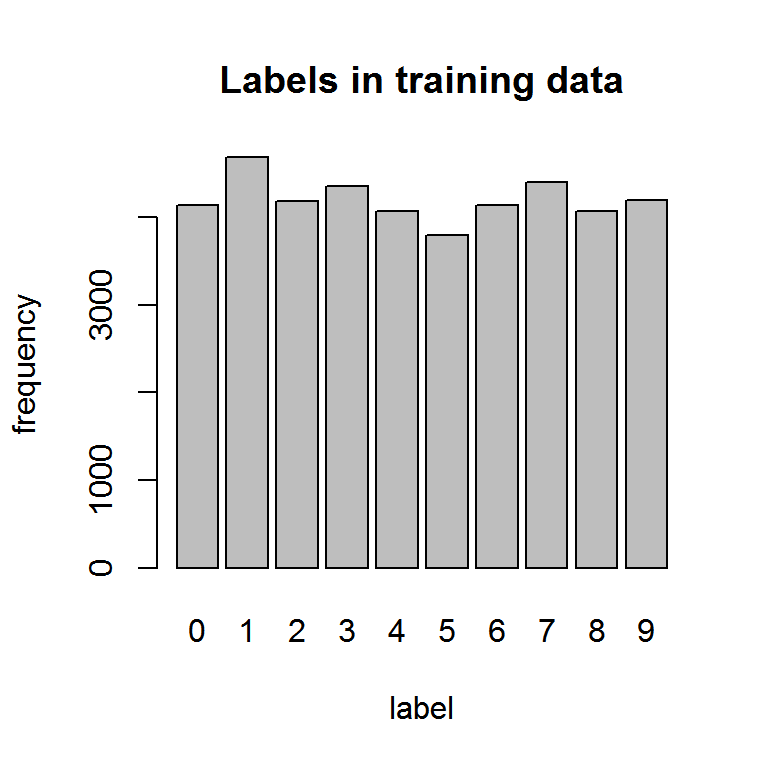Next I want to plot feature vectors for each type of digit. par(mar=c(1,1,1,1)) par(mfrow = c(2, 5)) for(imageLabel in levels(trainData$label)){
imageIndex <- which(trainData$label == imageLabel) #index of image of asked label image_features <- as.numeric(trainData[imageIndex, trainFeatureIndexes]) plot(image_features,asp=1) }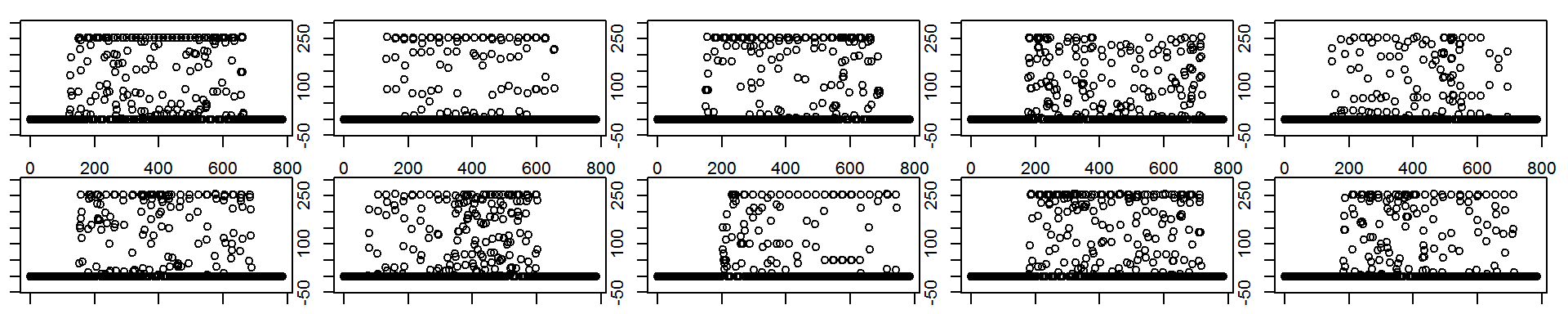The features actually represent images and I can transform them to images. plotImageVector <- function(image_features){ fix <- function(m){ t(m)[,nrow(m):1] } as_image <- fix(matrix(image_features, nrow=imageSize, ncol=imageSize, byrow=TRUE)) image(255 - as_image, axes = FALSE, col = grey(seq(0, 1, length = 256)),asp=1) } plotImage <- function(imageIndex){ imageFeatures <- as.numeric(trainData[imageIndex, trainFeatureIndexes]) plotImageVector(imageFeatures) } par(mar=c(1,1,1,1)) par(mfrow = c(2, 5)) for(imageLabel in levels(trainData$label)){
imageIndex <- which(trainData$label == imageLabel) #index of image of asked label plotImage(imageIndex) }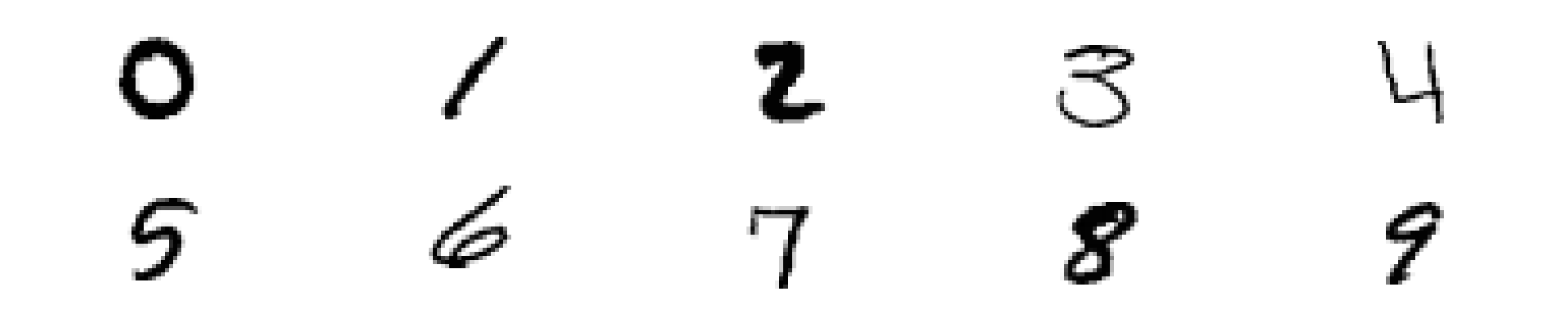Next, I want to browse the data per class (per digit). I use kmeans to extract a number of prototypes for each digit. (Although one could do better than kmeans this is sufficient for this dataset.) showPrototypes <- function(imageLabel, numProt){ idx <- which(trainData$label == imageLabel) #select indexes
#0.0 forces conversion from integer to numeric
subData <- 0.0 + data.matrix(trainData[idx, trainFeatureIndexes])
res <- kmeans(subData, centers = numProt)
centers <- res$centers numReturnedCenters <- dim(centers) for(i in seq(1, numReturnedCenters)){ centerI = centers[i, ] plotImageVector(centerI) } #for(i in seq(numReturnedCenters + 1, numProt)){ #plot(c()) #empty plot if necessary #} } par(mar=c(1,1,1,1)) #10 rows and 5 columns par(mfrow = c(length(levels(trainData$label)), 5))
for(imageLabel in levels(trainData$label)){ showPrototypes(imageLabel, 5) } ## Warning: did not converge in 10 iterations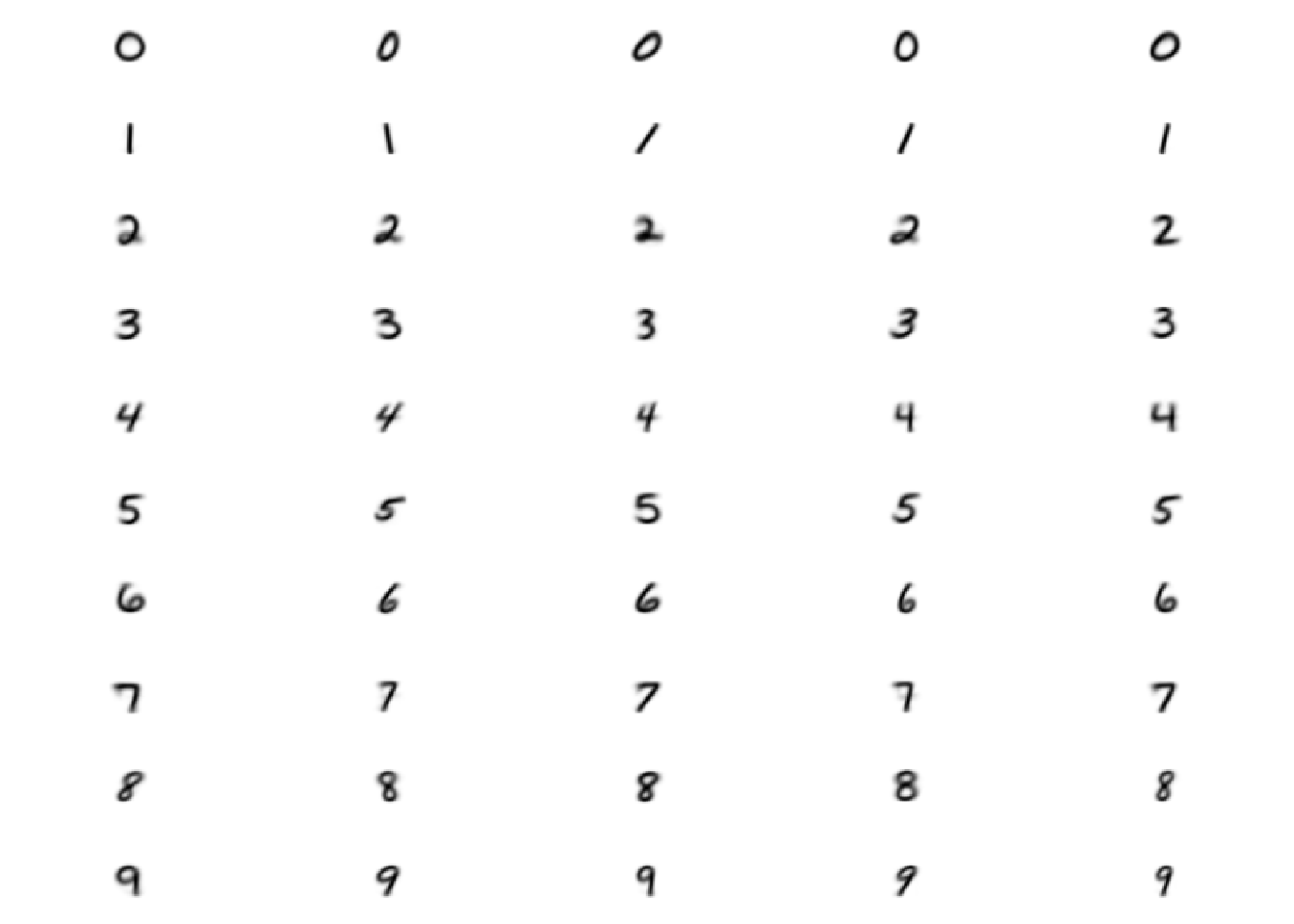What happens, if I cluster the full dataset into 20 prototypes. I see at least one prototype per digit. That’s good! numProt = 20 fullData <- 0.0 + data.matrix(trainData[, trainFeatureIndexes]) res <- kmeans(fullData, centers = numProt) ## Warning: did not converge in 10 iterations centers <- res$centers
numReturnedCenters <- dim(centers)
par(mar=c(1,1,1,1))
#10 rows and 2 columns
par(mfrow = c(10, 2))
for(i in seq(1, numReturnedCenters)){
centerI = centers[i, ]
plotImageVector(centerI)
}